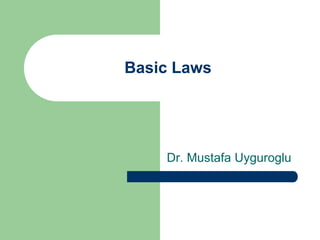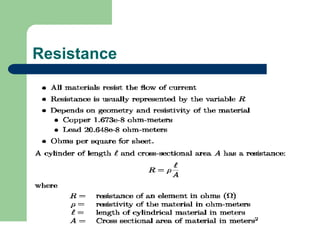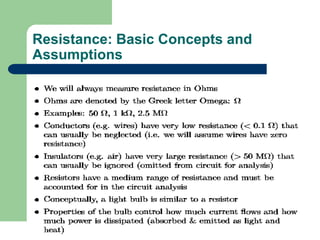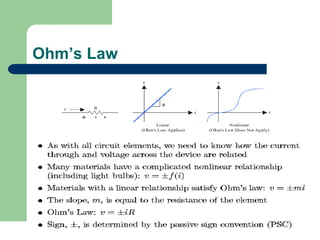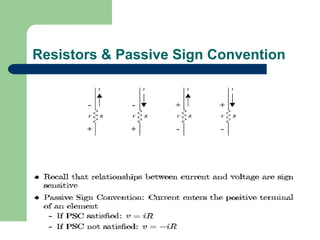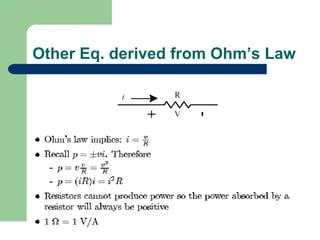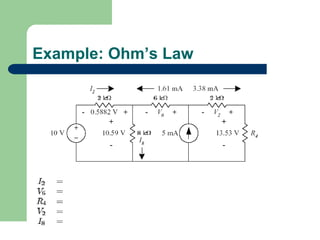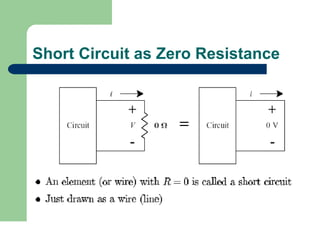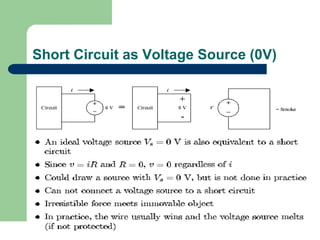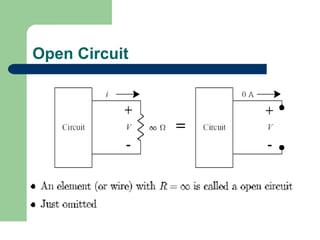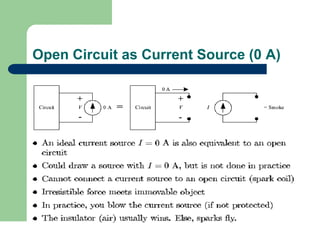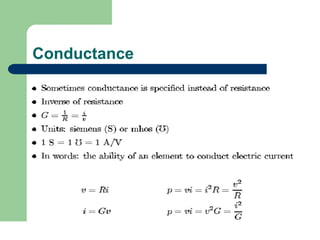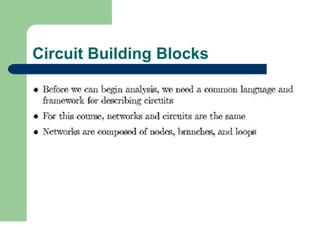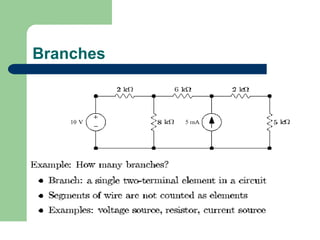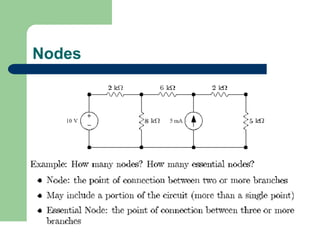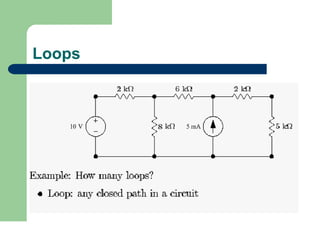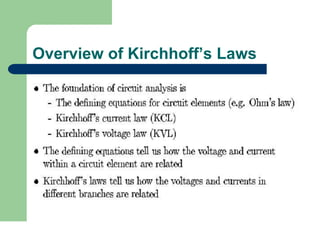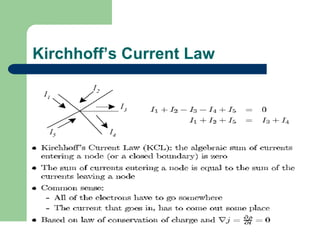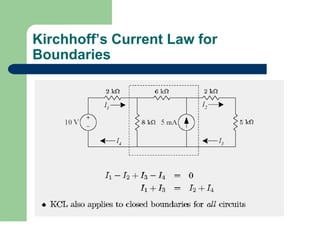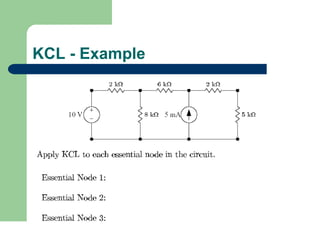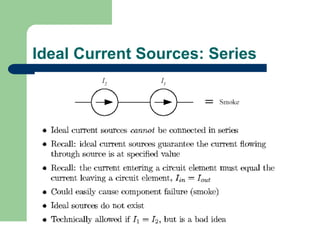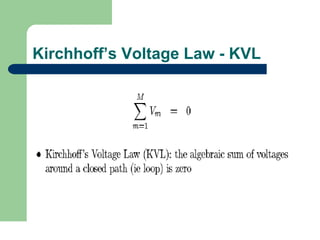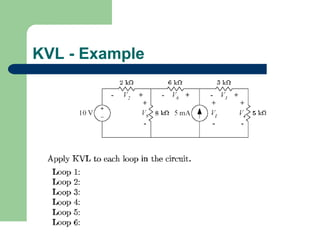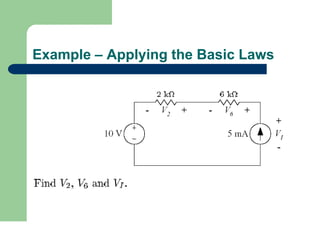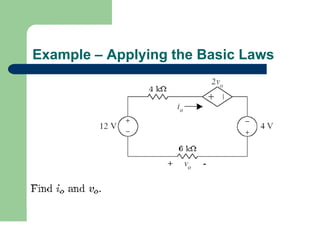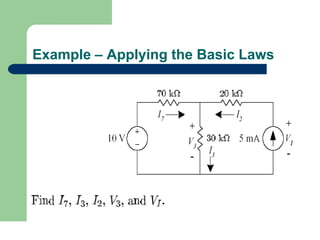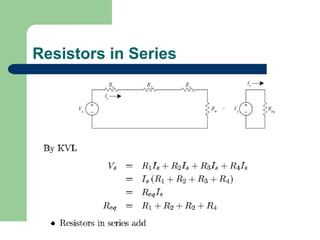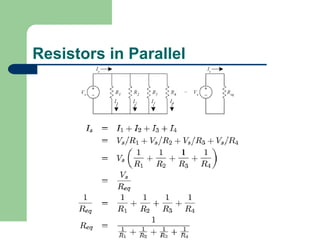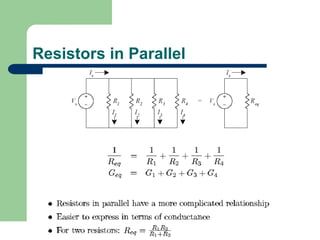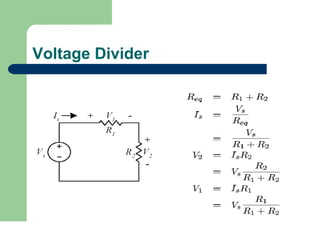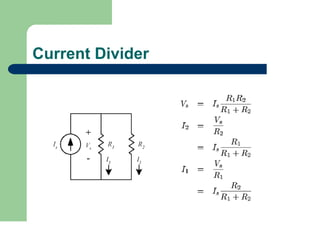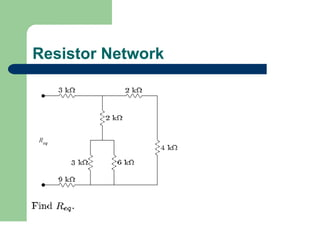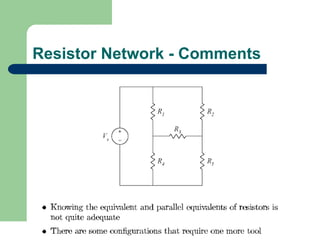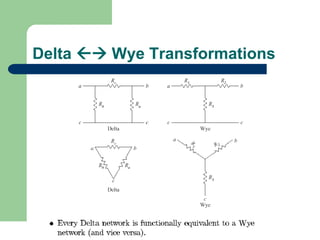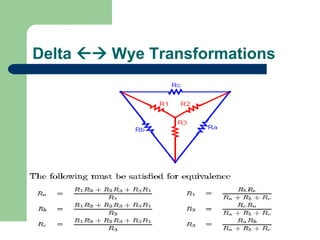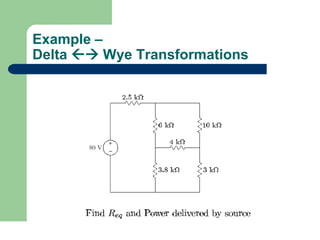1 of 36

### Basic_Laws.ppt

1. Basic Laws Dr. Mustafa Uyguroglu
2. Resistance
3. Resistance: Basic Concepts and Assumptions
4. Ohm’s Law
5. Resistors & Passive Sign Convention
6. Other Eq. derived from Ohm’s Law
7. Example: Ohm’s Law
8. Short Circuit as Zero Resistance
9. Short Circuit as Voltage Source (0V)
10. Open Circuit
11. Open Circuit as Current Source (0 A)
12. Conductance
13. Circuit Building Blocks
14. Branches
15. Nodes
16. Loops
17. Overview of Kirchhoff’s Laws
18. Kirchhoff’s Current Law
19. Kirchhoff’s Current Law for Boundaries
20. KCL - Example
21. Ideal Current Sources: Series
22. Kirchhoff’s Voltage Law - KVL
23. KVL - Example
24. Example – Applying the Basic Laws
25. Example – Applying the Basic Laws
26. Example – Applying the Basic Laws
27. Resistors in Series
28. Resistors in Parallel
29. Resistors in Parallel
30. Voltage Divider
31. Current Divider
32. Resistor Network